×

Search anything:

# Minimum Cut Problem [Overview]

#### Algorithms Graph AlgorithmsGet this book -> Problems on Array: For Interviews and Competitive Programming

In this article, we have covered the basics of Minimum Cut Problem, its applications like Network Reliability, algorithms like Ford-Fulkerson Algorithm to solve it and much more.

# Problem Definition

In order to understand the Minimum Cut Problem (often abbreviated to Min-Cut Problem), we first need to define a "Cut".

### Cut

A Cut in a graph G is defined as the partition of the vertices in a graph into two disjoint proper subsets.
More formally, consider a graph $G=(V, E)$:
A cut $C = (S, T)$ partitions $V$ into two proper disjoint subsets $S,T$ such that $\{(u,v) \in E | u \in S, v \in T\}$ and $C \neq \emptyset, V$ (i.e $C \subset V$).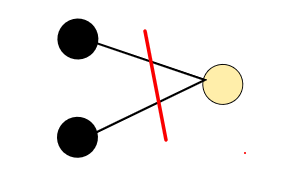Cut Size = 2

In an unweighted undirected graph, the size of a cut is the number of edges crossing the cut between $S$ and $V \setminus S$, whereas in a weighted graph it is the sum of the weights that cross the cut.

## Minimum Cut

A minimum cut is defined as a cut that is minimal in either the number of edges that cross the cut (unweighted) or the weights of the edges that cross the cut (weighted). More formally, it is defined as $\{min(|C|) : \emptyset \neq C \subset V\}$

## Minimum Cut Problem

In order to define the Min-Cut problem, it is important to note that there are essentially two different variations of the problem.

The first variation is know as the "Minimum s-t Cut Problem" and is defined as follows:

Input: Undirected graph $G= (V, E)$, with vertices $s$ and $t$
Output: A partition of graph G into two proper disjoint subsets $V$ and $S$ such that $s \in S$ and $t \in V$ and the number of edges crossing the cut is minimized.

The second variation can be thought of as a "global" version of the first, where no terminal nodes are given, and we are simply looking for the minimum cut across all pairs of vertices $s$ and $t$.

The Minimum Cut Problem is defined as follows:
Input: An undirected graph $G=(U,V)$
Output: A minimal set M of edges that cross a cut

## Applications

It is often useful to first explore the applications of such a problem in order to motivate the need for finding an efficient and useful solution.

### Network Reliability

We can use an efficient solution to the minimum cut problem in order to
estimate the probability of a network failure. Note that a network failure will occur if our network becomes disconnected. Our definition of a cut as stated above indicates that if the set of edges that compose the cut were to be removed, or in the case of a network, fail, the graph (network) would become disconnected. Assuming all edges have an equal probability of failing, it is clear to see that the cut with the least amount of edges is most likely to become disconnected, and therefore most likely to cause network failure.

### Image Segmentation

Image segmentation is the partitioning of images into disjoint sets of pixels such that pixels with similar characteristics are grouped together, often with the goal of simplifying an image. Consider a graph $G$ where the vertices $V$ represent pixels, and the edges $E$ represent relationships between similar pixels. In this case, the solution to the Min-Cut problem provides use with the partition of pixels where the two sets are the most dissimilar.

## Algorithms

Now that we understand the importance of the Min-Cut problem, it is time we consider some potential solutions.

We will first consider solutions to the "Minimum s-t Cut Problem". Despite the multiple potential algorithms to this specific problem, they all utilize a fundamental theorem known as the Max-Flow Min-Cut theorem. In the following definition, flow refers to the weight of an edge (in the case of an unweighted graph we can assume the weight to be 1).

## Max-Flow Min-Cut theorem:

The Minimum s-t Cut is equal to the Maximum Flow for any graph $G$.

The above theorem tell us that a solution to one of the problems will provide us a solution to the other. Keeping this in mind, we can breifly analyze the following Maximum Flow Algorithm.

## Ford-Fulkerson Algorithm

The Ford-Fulkerson is a greedy algorithm that computes the maximum flow of a network (which as shown above provides use with a solution to the Min s-t Cut Problem).

The main idea of the algorithm is as follows:
Let F denote the flow and C denote the capacity of an arbitrary but particular edge $(u,v) \in G$

1. Let F(u,v) = 0 for all edges (u,v) in E
2. While there is an augmented path from s to t:
F(u,v) = F(u,v) + (C(path) - F(path))
F(v,u) = F(v,u) - (C(path) - F(path))
3. Return F(s,t)

Where the path in step 2 can be found using BFS or DFS, and $F(s,t) =$ Min-Cut s-t by the Max-Flow Min-Cut theorem.

Complexity: Assuming the flow values of each of the edges is an integer, the runtime is given by $O(EF)$ where E is the number of edges and F is the maximum flow. It is worth noting that if the flow values of any of the edges are irrational, the algorithm is not guaranteed to terminate. That being said the Edmonds-Karp algorithm, a variation of the above algorithm that enforces the use of BFS, can be used to find an answer if there exists irrational numbers in $O(VE^2)$ time.

We will now analyze a potential solution for finding the global Min-Cut (i.e
when no terminal vertices are given).

## Brute Force

The naive approach would be to fix a node $s$, and for each remaining vertex $t$, use the above algorithm to find the Max Flow (Min-Cut) between these pairs, and return this minimum of all iterations. The returned pair would be the minimum global cut since we iterate through all pairs $s,t$ and the global minimum cut must exist for some nodes s and t. This would result in the above algorithm running for $|V| - 1$ times, which is not suitable for larger graphs.

## Kargers Algorithm

Kargers Algorithm is specific type of randomized algorithm known as a "Monte Carlo" algorithm (not to be confused with "Las Vegas" algorithm). Essentially, the algorithms runtime is bounded by a function in the size of the input, however the algorithm may return an incorrect answer with small probability.

The algorithm defines "supernodes" and "superedges". A supernode is a group of nodes. A superedge is an edge connecting two supernodes that consists of all edges between a pair of nodes in separate super nodes. Kargers randomly chooses edges and performs edge contractions. The procedure for an edge contraction is as follows:

2. For each edge x,u or u,x add the edge x,S
3. Every edge with endpoint u or v is removed
4. u and v are safely deleted from the graph

We can then define Kargers with the following procedure:

1. While there are at least 2 vertices:
Choose edge u,v uniformly at random from E
Contract into super vertex S using above procedure
2. Return the cut between the two remaining supernodes

Example: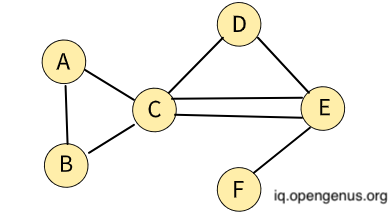A graph G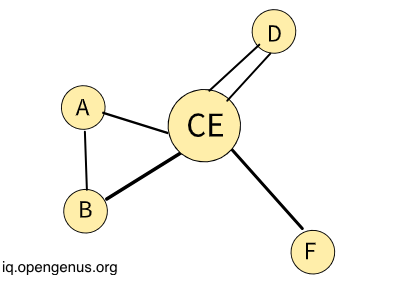Contracting edge C,E and E,C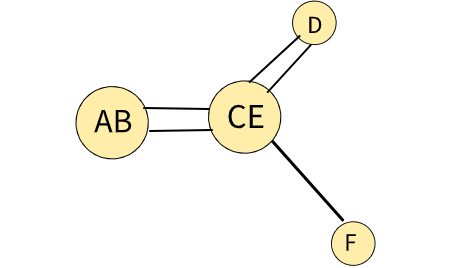Contracting edge A,B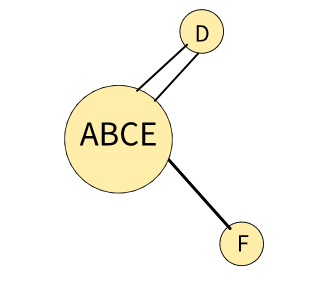Contracting edge CE,D and D,CE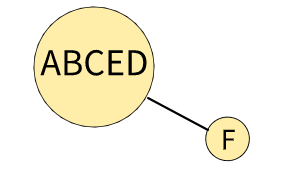Contracting edge ABCE,D and D,ABCE
Therefore Min-Cut = 1

Complexity: Assuming the graph $G$ is implemented using an adjacency list or matrix, the total running time is $O(|V|^2)$

Recall that Kargers is a Monte Carlo algorithm, therefore the probability that Kargers returns a min cut is at least $\frac{1}{n \choose 2}$

## Summary

1. A Cut in a graph $G$ is defined as the partition of the vertices in a graph into two disjoint subsets.

2. A minimum cut is defined as a cut that is minimal in either the number of edges that cross the cut (unweighted) or the weights of the edges that cross the cut (weighted).

3. The first variation is known as the "Minimum s-t Cut Problem".
The second variation is known as the "Global Minimum Cut Problem".

4. Min-Cut Max-Flow theorem allows us to use the answer from one as the answer to the other.

5. Can use Ford-Fulkerson Algorithm for s-t problem, and Krugals for global problem.

6. Solutions to the minimum cut problem are useful in predicting network
failure, image segmentation, and many other applications.

## Understanding Question 1

#### A cut is defined as

the partition of the vertices in a graph into two disjoint proper subsets
the partition of the vertices in a graph into two disjoint subsets
the partition of the edges in a graph into two disjoint proper subsets
the partition of the vertices in a graph into three subsets
Recall that a cut must not only be a partition of vertices into two disjoint subsets, but those subsets must also be proper! (i.e $C \neq \emptyset, V$)

## Understanding Question 2

#### Kargers Algorithm is known as a

Monte Carlo algorithm
Las Vegas algorithm
Roulette algorithm
Casino algorithm
Krugals is a Monte Carlo algorithm since its runtime is bounded in the size of input, but terminates with a small probability of having an incorrect answer.

#### Nolan Biscaro

SWE Intern at Bloomberg + BSE in Computer Science from University of Pennsylvania